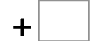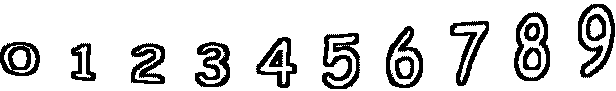7= 15   1= 67= 10   3= 5   9= 17   3= 10 2= 7   0= 3   5= 10   0= 10